﻿ Menu | Function-Block | Graph

# Function-Block | GraphKinematics FB toolbar > Graph FB

 STEP 1: Add a Graph FB to the graphic-area

- or -

Kinematics FB toolbar > Add Graph FB - see Kinematics Function-Block toolbar

 2 Click in the graphic-area

The Graph FB is now in the graphic-area.

 STEP 2: Connect wires
 1 Drag wires from the output-connectors of FBs to input-connectors of the Graph FB.

There are five(5) input-connectors on the Graph FB.

Counting the input-connectors from the Top of the Graph GB

 • #1 – 4 : Y-axes of the graph. - See Below: Y-axis Options
 • #5 : X-axis of the graph - See below X-axis Options

Note: Optional inputs to the X-Axis input-connector [#5 input-connector]

Option 1: No Wire - the X-axis becomes the same as the Master Machine Axis

Option 2: Linear-Motion FB - input motion-values increases linearly, the X-axis can thus have a scale that is not 0 – 360.

Option 3: From a Motion FB or Measurement FB - input motion-values does not increase at a constant rate.

Note: the graph may 'fly-back' from across from the end to the start of the plot if the input does not have a wire.

 STEP 3: Open the Graph FB
 1 Click the Graph FB in the graphic-area

The Graph FB is in the Selection-Window

 2 Right-click the Graph FB in the Selection-Window
 3 Click 'Edit element...' in the contact-sensitive menu.

or, see How to open a Dialog-Box

See: Graph FB to review the Graph Interface.

to review the kinematic, kinetostatic and Cam-Data that you can plot with a Graph FB.

####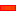Graph FB InterfaceThe Graph FB: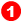Y–axis : Scales:

 • Maximum = 4, scales correspond to wires at input, Y1 to Y4
 • The colour of the Y–axis and the plot-line are the same.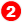Y–axis : Data-Channel Selector

 • Each wire that is connected to an input-connector has up to 3 Data-Channels
 • Select the Data-Channel in the drop-down box - see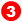Digital Readout (DRO):

 • The X–axis and the Y1 – Y4-axis values at the vertical cursor.
 • The Number-Format for the X–axis, Y–axis, and Data in Clipboardis configured in Application Settings | Number Format tab.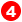The Graph Area:

 • The colour-coded graphs for each Y–axis input
 • Vertical-CursorThe Vertical-Cursor AND the Master Machine Angle 'jump' to where you click in the graph area, and also to where you drag your mouse pointer.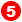Toolbar:

 • Save, Print, Graph Settings, Zoom Extents, Pan Left, Pan Right, 'Copy Graph Data to Clipboard' – seebelow

Also, note these tools: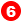Vertical-Cursor

When you click in the Graph area, a Vertical Cursor will snap to your mouse pointer

When you drag your pointer in the graphic-area:

 • the Vertical Cursor will move with your pointer along the X-axis
 • the Digital Readoutswill continuously update to indicate the Y-axis values at the X-axis
 • the Master Machine Angle will update to the value of the X-axis at the position of the Vertical Cursor
 • the kinematically-defined Chains will move to value of the Master Machine Angle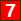Copy Graph Data to Clipboard

 • When you click the right-most tool/icon in the toolbar, above the Graphs, all of the graph data is copied to the Clipboard.

Notes:

 • Configure the Number-Format for the X–axis, Y–axis, and 'Data in Clipboard' in Application Settings | Number Format tab.
 • Paste this data to Excel, or to MotionDesigner Data Transfer table. You can plot it as an overlay or use it as a Motion [use Z Raw-Data].

####Y-Axis Data-Channel Options:

Each wire you connect to the Graph FB has three Data-Channels.

Use the Y-axis Selector to select which data-channel to plot.

The data-channels that are available with each wire are a function of the FB from which you connector the wire to the Graph FB input-connector.

If the wire you connect to the Y–axis input-connector is from a:

 • Function-Block (Linear-Motion, Gearing, Motion, Motion-Dimension):
 o Linear position, velocity, acceleration of the Measurement Dimension or Point.
 o Angular position, velocity, acceleration of the Measurement Dimension.
 • Measurement FB or Point-Data FB:
 o Linear position, velocity, acceleration of the Measurement or Point
 o Angular position, velocity, acceleration of the Measurement
 • Cam-Data FB
 o Pressure-Angle. You can plot three Pressure Angles – See Pressure Angle
 o Cam Radius-of-Curvature. You can plot Radii-of-Curvature for Cam 1 and Cam 2.
 o Contact Cam-Force
 o Maximum Contact-Shear-Stress. You must know the material-properties to obtain this data. See Cam Display and Properties dialog-box.
 • Force-Data FB

If the Force-Data FB references aÖ

 o Pin-Joint with a motor, and gearbox  you can plot Motor Drive Torque.
 o Slide-Joint with a motor, you can plot Motor Drive Linear Force
 o Joint
 ▪ Total Force [ FT = √(FX2 + FY2), or
 ▪ Force in the X-axis direction : FX
 ▪ Force in the Y-axis direction: FY
 o 2D-Cam: you can plot the Cam Contact Force
 o Spring FB: you can plot the Total Force, the X Force and the Y Force acting on the Point.

Note:

To show all three Data-Channels from one output-connector a Function-Block, then drag a wire from the same output-connector to different input-connectors on the Graph FB.

Tutorial and Reference Help Files for MechDesigner and MotionDesigner 13.2 + © Machine, Mechanism, Motion and Cam Design Software by PSMotion Ltd